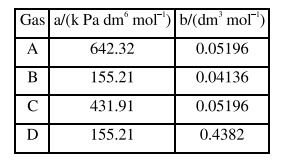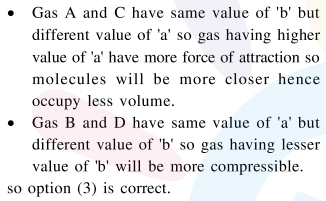Deepak Scored 45->99%ile with Bounce Back Crack Course. You can do it too!

# Consider the following table :

Question:

Consider the following table :a and $b$ are vander waals constant. The correct statement about the gases is :

1. Gas C will occupy lesser volume than gas A; gas B will be lesser compressible than gas D

2. Gas $C$ will occupy more volume than gas A; gas B will be lesser compressible than gas D

3. Gas C will occupy more volume than gas $A$; gas $B$ will be more compressible than gas D

4. Gas $C$ will occupy lesser volume than gas $A$; gas $\mathrm{B}$ will be more compressible than gas D

Correct Option: , 3

Solution: